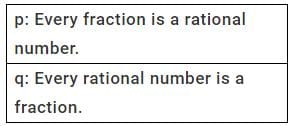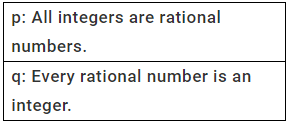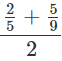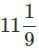Important Questions: Rational Numbers

# Important Questions: Rational Numbers

Test Description

## 20 Questions MCQ Test Mathematics (Maths) Class 7 | Important Questions: Rational Numbers

Important Questions: Rational Numbers for Class 7 2022 is part of Mathematics (Maths) Class 7 preparation. The Important Questions: Rational Numbers questions and answers have been prepared according to the Class 7 exam syllabus.The Important Questions: Rational Numbers MCQs are made for Class 7 2022 Exam. Find important definitions, questions, notes, meanings, examples, exercises, MCQs and online tests for Important Questions: Rational Numbers below.
Solutions of Important Questions: Rational Numbers questions in English are available as part of our Mathematics (Maths) Class 7 for Class 7 & Important Questions: Rational Numbers solutions in Hindi for Mathematics (Maths) Class 7 course. Download more important topics, notes, lectures and mock test series for Class 7 Exam by signing up for free. Attempt Important Questions: Rational Numbers | 20 questions in 20 minutes | Mock test for Class 7 preparation | Free important questions MCQ to study Mathematics (Maths) Class 7 for Class 7 Exam | Download free PDF with solutions
 1 Crore+ students have signed up on EduRev. Have you?
Important Questions: Rational Numbers - Question 1

###Which of the following is correct?

Detailed Solution for Important Questions: Rational Numbers - Question 1

Let us consider a fraction 3/5 and a rational number = 14/7
By definition, 3/5 is a rational number. So, p is true. By definition, -4/7 is not a fraction, since, −4 is not a natural number. So, q is false.

All fractions can be termed as rational numbers; however, all rational numbers cannot be termed as fractions. Only those rational numbers in which 'p' and 'q' are positive integers are termed as fractions.

Important Questions: Rational Numbers - Question 2

### Which of the following is not a rational number(s)?

Detailed Solution for Important Questions: Rational Numbers - Question 2

√2/3 is not a rational number.

because √2 doesn't have an exact value, and on further division by 3, it goes more unstable.

Important Questions: Rational Numbers - Question 3

###Which of the following statements is correct?

Detailed Solution for Important Questions: Rational Numbers - Question 3

Since, every integer having a denominator 1 can be expressed in P/q form, p is true. Since, a rational number with denominator other than 1 is not an integer (e.g., 3/5), q is false.

Important Questions: Rational Numbers - Question 4

What type of a number is −3/0 ?

Detailed Solution for Important Questions: Rational Numbers - Question 4

Since, denominator is 0, it is not a rational number.

Important Questions: Rational Numbers - Question 5

Which among the following is a rational number equivalent to 5/3?

Important Questions: Rational Numbers - Question 6

What type of a numerator does 0/7 have?

Important Questions: Rational Numbers - Question 7

Which of the following statements is true?

Detailed Solution for Important Questions: Rational Numbers - Question 7

-12/32 = -3*4/8*4 = -3/8

Important Questions: Rational Numbers - Question 8

If −4/7 = −32/x, what is the value of x?

Detailed Solution for Important Questions: Rational Numbers - Question 8

-4/7 = -32/X

X=32x7/4

X=56

Important Questions: Rational Numbers - Question 9

Which is the greatest?

Important Questions: Rational Numbers - Question 10

Which is the correct descending order of −2, 4/−5, −11/20, 3/4?

Detailed Solution for Important Questions: Rational Numbers - Question 10

L.C.M. of 5, 4 and 20 is 20.
4/−5 × 4/4 = 16/−20;3/4 = 3×5/4×5 = 15/20
−2 < 4/−5 < −11/20 < 3/4
or
3/4 > −11/20 > 4/−5 > −2
is the required descending order.
Rewrite equivalent fractions of the given fractions and then compare then.
Arrange them in descending order.

Important Questions: Rational Numbers - Question 11

What is the average of the two middle rational numbers if 4/7,1/3,2/5 and 5/9 are arranged in ascending order?

Detailed Solution for Important Questions: Rational Numbers - Question 11

The ascending order of 4/7,1/3, 2/5 and 5/9 is 1/3<2/5<5/9<4/7. The two middle numbers are 2/5 and 5/9.

∴  Average == 43/90

Important Questions: Rational Numbers - Question 12

What is the percentage of the least number in the greatest number of 3/5,9/5,1/5 and 7/5 ?

Detailed Solution for Important Questions: Rational Numbers - Question 12

The given numbers can be arranged in ascending order as1/5 < 3/5 < 7/5 < 9/5
The greatest number = 9/5;
The least number = 1/5
We have,  9/5 × x/100 = 1/5
⇒ x = 10/09 =Important Questions: Rational Numbers - Question 13

What is the difference between the greatest and least numbers of 5/9,1/9 and 11/9 ?

Detailed Solution for Important Questions: Rational Numbers - Question 13

The ascending order of given numbers is 1/9,5/9,11/9.
∴  Required difference = 11/9 −1/9 = 10/9

Important Questions: Rational Numbers - Question 14

Which of the following pairs represent the same rational number?

Detailed Solution for Important Questions: Rational Numbers - Question 14

−24/15 is the equivalent rational number of −8/5.

Important Questions: Rational Numbers - Question 15

P: The quotient of two integers is always a rational number.
Q : 1/0 is not rational. Which of the following statements is true?

Detailed Solution for Important Questions: Rational Numbers - Question 15

Since, 1/0 is not rational, the quotient of two integers is not always rational.

Important Questions: Rational Numbers - Question 16

What is the result of 2 − 11/39 + 5/26?

Important Questions: Rational Numbers - Question 17

Which is the equivalent of −143/21?

Important Questions: Rational Numbers - Question 18

Of which property is −7/5 + (2/−11 + −13/25) = (−7/5 + 2/−11) + −13/25 an example?

Important Questions: Rational Numbers - Question 19

Which of the following statements is correct?

Detailed Solution for Important Questions: Rational Numbers - Question 19

All the given statements are correct. Consider
4/5 [a] Since 4/5 + 0 = 4/5,, 1 is the additive identity of rational numbers. [b] Since
4/5 × 1= 4/5, is the  multiplicative identity of rational numbers. [c] Since 0+0=0,
0 is the additive inverse of 0.

Important Questions: Rational Numbers - Question 20

The sum of two rational numbers is −3. If one of the numbers is −7/5, find the other number.

Detailed Solution for Important Questions: Rational Numbers - Question 20

Let x be the required number. So,
x+(−7/5) = −3
⇒ x = −3+7/5 = −1/5 + 7/5 = −8/5

## Mathematics (Maths) Class 7

168 videos|252 docs|45 tests
 Use Code STAYHOME200 and get INR 200 additional OFF Use Coupon Code
Information about Important Questions: Rational Numbers Page
In this test you can find the Exam questions for Important Questions: Rational Numbers solved & explained in the simplest way possible. Besides giving Questions and answers for Important Questions: Rational Numbers, EduRev gives you an ample number of Online tests for practice

## Mathematics (Maths) Class 7

168 videos|252 docs|45 tests